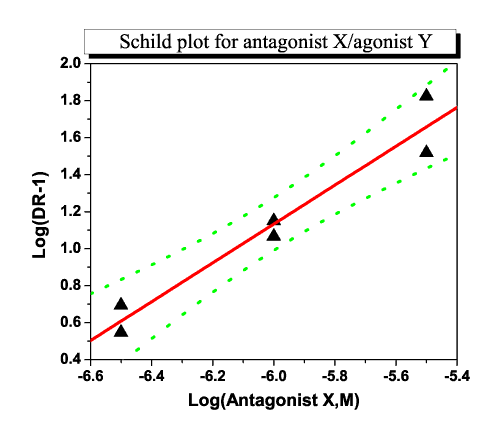Skip Navigation Links.

Schild Plot Showing Results of Linear RegressionThis Schild Plot shows the results of a linear regression (plotted as a red line plot) that was performed on some raw data (plotted as a scatter plot using solid, black triangles). The green line plots above and below the regression line represent the 95% confidence bands. A title with a shadow background has been added, as well as a line opposite each axis that enclose the graph for presentation purposes.